## Huygens’s Principle: Diffraction

### Learning Objectives

By the end of this section, you will be able to:

• Discuss the propagation of transverse waves.
• Discuss Huygens’s principle.
• Explain the bending of light.

Figure 1 shows how a transverse wave looks as viewed from above and from the side. A light wave can be imagined to propagate like this, although we do not actually see it wiggling through space. From above, we view the wavefronts (or wave crests) as we would by looking down on the ocean waves. The side view would be a graph of the electric or magnetic field. The view from above is perhaps the most useful in developing concepts about wave optics.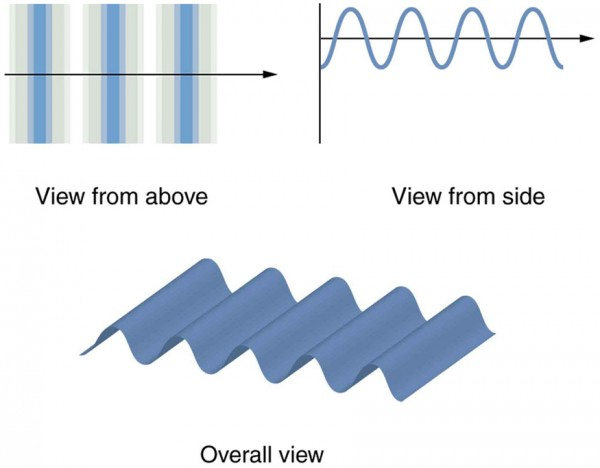Figure 1. A transverse wave, such as an electromagnetic wave like light, as viewed from above and from the side. The direction of propagation is perpendicular to the wavefronts (or wave crests) and is represented by an arrow like a ray.

The Dutch scientist Christiaan Huygens (1629–1695) developed a useful technique for determining in detail how and where waves propagate. Starting from some known position, Huygens’s principle states that:

Every point on a wavefront is a source of wavelets that spread out in the forward direction at the same speed as the wave itself. The new wavefront is a line tangent to all of the wavelets.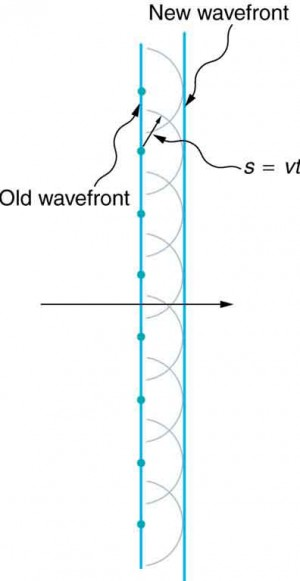Figure 2. Huygens’s principle applied to a straight wavefront. Each point on the wavefront emits a semicircular wavelet that moves a distance . The new wavefront is a line tangent to the wavelets.

Figure 2 shows how Huygens’s principle is applied. A wavefront is the long edge that moves, for example, the crest or the trough. Each point on the wavefront emits a semicircular wave that moves at the propagation speed v. These are drawn at a time t later, so that they have moved a distance svt. The new wavefront is a line tangent to the wavelets and is where we would expect the wave to be a time t later. Huygens’s principle works for all types of waves, including water waves, sound waves, and light waves. We will find it useful not only in describing how light waves propagate, but also in explaining the laws of reflection and refraction. In addition, we will see that Huygens’s principle tells us how and where light rays interfere.

Figure 3 shows how a mirror reflects an incoming wave at an angle equal to the incident angle, verifying the law of reflection. As the wavefront strikes the mirror, wavelets are first emitted from the left part of the mirror and then the right. The wavelets closer to the left have had time to travel farther, producing a wavefront traveling in the direction shown.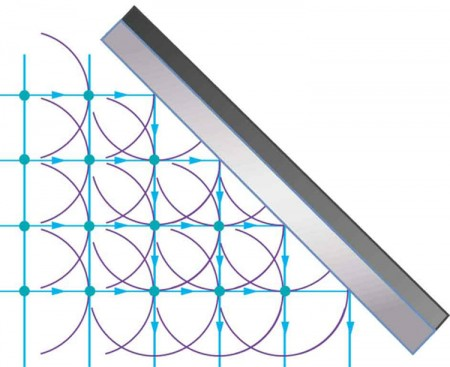Figure 3. Huygens’s principle applied to a straight wavefront striking a mirror. The wavelets shown were emitted as each point on the wavefront struck the mirror. The tangent to these wavelets shows that the new wavefront has been reflected at an angle equal to the incident angle. The direction of propagation is perpendicular to the wavefront, as shown by the downward-pointing arrows.

The law of refraction can be explained by applying Huygens’s principle to a wavefront passing from one medium to another (see Figure 4). Each wavelet in the figure was emitted when the wavefront crossed the interface between the media. Since the speed of light is smaller in the second medium, the waves do not travel as far in a given time, and the new wavefront changes direction as shown. This explains why a ray changes direction to become closer to the perpendicular when light slows down. Snell’s law can be derived from the geometry in Figure 4, but this is left as an exercise for ambitious readers.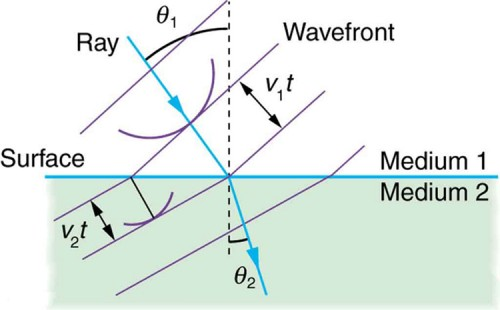Figure 4. Huygens’s principle applied to a straight wavefront traveling from one medium to another where its speed is less. The ray bends toward the perpendicular, since the wavelets have a lower speed in the second medium.

What happens when a wave passes through an opening, such as light shining through an open door into a dark room? For light, we expect to see a sharp shadow of the doorway on the floor of the room, and we expect no light to bend around corners into other parts of the room. When sound passes through a door, we expect to hear it everywhere in the room and, thus, expect that sound spreads out when passing through such an opening (see Figure 5). What is the difference between the behavior of sound waves and light waves in this case? The answer is that light has very short wavelengths and acts like a ray. Sound has wavelengths on the order of the size of the door and bends around corners (for frequency of 1000 Hz, $\lambda=\frac{c}{f}=\frac{330\text{ m/s}}{1000\text{ s}^{-1}}=0.33\text{ m}\\$, about three times smaller than the width of the doorway).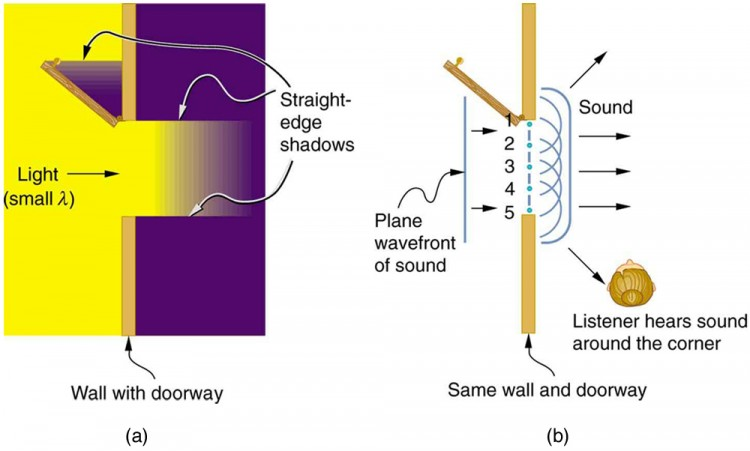Figure 5. (a) Light passing through a doorway makes a sharp outline on the floor. Since light’s wavelength is very small compared with the size of the door, it acts like a ray. (b) Sound waves bend into all parts of the room, a wave effect, because their wavelength is similar to the size of the door.

If we pass light through smaller openings, often called slits, we can use Huygens’s principle to see that light bends as sound does (see Figure 6). The bending of a wave around the edges of an opening or an obstacle is called diffraction. Diffraction is a wave characteristic and occurs for all types of waves. If diffraction is observed for some phenomenon, it is evidence that the phenomenon is a wave. Thus the horizontal diffraction of the laser beam after it passes through slits in Figure 7 is evidence that light is a wave.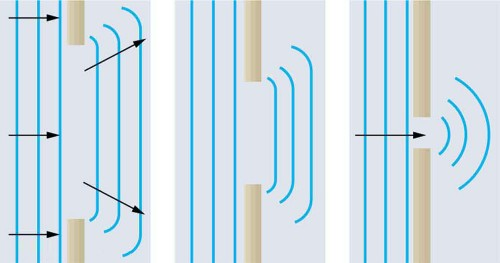Figure 6. Huygens’s principle applied to a straight wavefront striking an opening. The edges of the wavefront bend after passing through the opening, a process called diffraction. The amount of bending is more extreme for a small opening, consistent with the fact that wave characteristics are most noticeable for interactions with objects about the same size as the wavelength.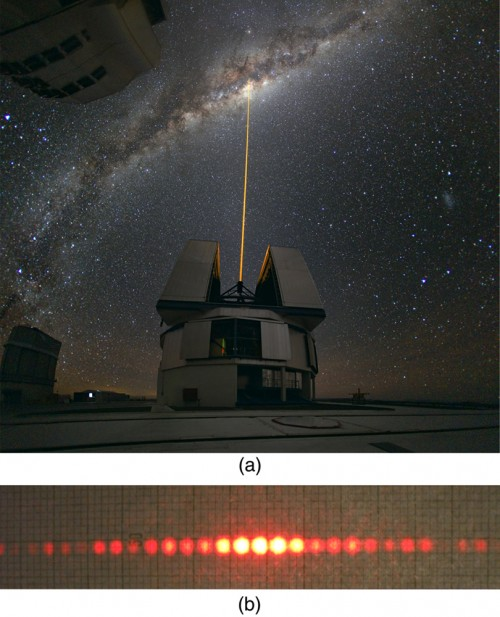Figure 7. (a) The laser beam emitted by an observatory acts like a ray, traveling in a straight line. This laser beam is from the Paranal Observatory of the European Southern Observatory. (credit: Yuri Beletsky, European Southern Observatory) (b) A laser beam passing through a grid of vertical slits produces an interference pattern—characteristic of a wave. (credit: Shim’on and Slava Rybka, Wikimedia Commons)

## Section Summary

• An accurate technique for determining how and where waves propagate is given by Huygens’s principle: Every point on a wavefront is a source of wavelets that spread out in the forward direction at the same speed as the wave itself. The new wavefront is a line tangent to all of the wavelets.
• Diffraction is the bending of a wave around the edges of an opening or other obstacle.

### Conceptual Questions

1. How do wave effects depend on the size of the object with which the wave interacts? For example, why does sound bend around the corner of a building while light does not?
2. Under what conditions can light be modeled like a ray? Like a wave?
3. Go outside in the sunlight and observe your shadow. It has fuzzy edges even if you do not. Is this a diffraction effect? Explain.
4. Why does the wavelength of light decrease when it passes from vacuum into a medium? State which attributes change and which stay the same and, thus, require the wavelength to decrease.
5. Does Huygens’s principle apply to all types of waves?

## Glossary

diffraction: the bending of a wave around the edges of an opening or an obstacle

Huygens’s principle: every point on a wavefront is a source of wavelets that spread out in the forward direction at the same speed as the wave itself. The new wavefront is a line tangent to all of the wavelets